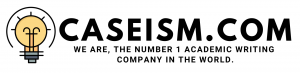# H. J. HEINZ Case Solution & Answer

## H. J. HEINZ Case Study Solution

Heinz uses a risk-free interest rate of 3.69% derived from the US Treasury rate. This means that Heinzâ€™s systemic risk is 3.69% and the investors cannot distribute it. The risk-free interest rate of 3.69% shows the value of money over time, which is the monetary value over time, which investors have to pay in order to invest in the companies or even the US market, because despite all the other risks involved; this risk cannot be diversified from Heinz or any collaboration of the company.

In the case of Heinz, 5.5% of the market risk premium was given, which represents the investorâ€™s demand for investing in the risk-free instruments rather than the non-risk-free instruments. In this case, a value of 0.62 was specified for the beta version. Heinzâ€™s expected return on equity is 7.10%, which shows that the Heinzâ€™s beta is relatively lower to the market or industry (less than 1) but provides a return to investors and shareholders.

### Table 2

 Capital Asset Pricing Model (CAPM) Expected return for cost of equity = rf+ B (rm-rf) rf = risk free rate = 3.69% (rm – rf) = market risk premium = 5.50% B = beta for Heinz = 0.62 Expected return for cost of equity = 7.10%

### Capital Structure

The firmâ€™s capital structure is 70% debt and 30% equity.

### Table 3

 Weight Short term debt = Â Â Â Â Â Â Â Â Â Â Â Â Â  43,853 0.67% LTD = Â Â Â Â Â Â Â Â Â  4,559,152 69.59% Equity = Â Â Â Â Â Â Â Â Â  1,948,496 29.74% Total = Â Â Â Â Â Â Â Â Â  6,551,501 100%

### WACC

The Heinzâ€™s WACC is 5.89%, which is comprised of 70% debt in the capital structure, and 30% equity in the capital structure. Although the cost of equity and the cost of debt in the WACC of Heinz during 2010, are:7.10% and 5.371%, respectively(Booth, Cleary,, & Drake, 2013).

### Table 4

 Weighted average cost of capital (WACC) WACC = Cost of equity * weight of equity + (cost of LTD + cost of STD) * Weighted Average Cost of Debt WACC = 5.89%

### Kraftâ€™s WACC:

The Kraftâ€™s WACC is 6.21%, which is comprised of 42% debt in the capital structure and 58% equity in the capital structure. Although, the cost of equity and the cost of debt in the Kraftâ€™s WACC during 2010, are: 8.04% and 5.12% respectively. The cost of equity is calculated by using a CAPM (Capital Asset Pricing Model) in which beta is given in exhibit 4 as 0.58. The risk-free rate and the average market premium are same as 3.69% and 7.5%, respectively.

### Campbellâ€™s WACC:

The Campbellâ€™s WACC is 3.79%, which is comprised of 78% debt in the capital structure and 22% equity in the capital structure. Although the cost of equity and the cost of debt after tax in the Campbellâ€™s WACC during 2010 are: 6.09% and 3%, respectively. The cost of equity is calculated by using a CAPM (Capital Asset Pricing Model) in which beta given in exhibit 4 is 0.32, which shows that company has lower risk portfolio. The risk-free rate and the average market premium are 3.69% and 7.5%, respectively.